Search IntMath
Close

# Pulleys interactive applet: math background

By Murray Bourne, 01 May 2014

I recently updated the pulleys interactive applet, so it now works on tablets. Before, it used Flash (which doesn't work on most mobile devices), but I rebuilt it using a javascript-based library (JSXGraph), so it works on tablets and phones.

As I developed it, I used quite a bit of interesting math.

I get quite a few mails from people asking how I develop these applets, so here is some mathematical background.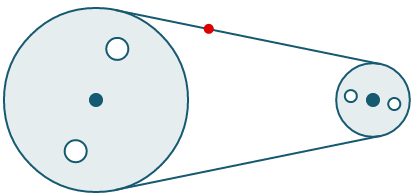## Analytic geometry

The 2 circles are built using the basic formula for a circle: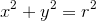The left-hand circle has center (0,0), (I could have placed it anywhere on the x-y plane, but (0,0) seemed simplest), and the radius it has at the start is 5. So its equation is: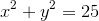The right hand circle has center (15,0) and radius 2 at the start.

If the circle has center other than the origin, say (h,k), we use this formula: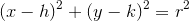So the equation of the right hand circle is: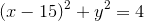[JSXGraph allows us to easily draw circles with different centers and different radii (without resorting to the formulas), but I still needed to make use of the formulas for later steps.]

I have hidden the axes for the applet to make it cleaner.

## Intersection points

The red dot follows the belt around. It travels through 2 circular arcs, and 2 straight line segments.

I needed to find the intersection point of the arcs with the straight line segments. This could be done using either Non-linear systems of equations or via other geometric constructions.

Of course these intersection points change when the radius of each wheel changes.

## Straight lines

I assumed no gravity was operating on the belt, and that it is (very) tight.

The red dot follows 2 line segments. I needed to find the equation of those segments. So I needed to use the basic equation for a straight line with slope m and y-intercept c: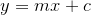To find the actual values of m and c, I needed to use the following formula, once I found the intersection points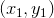and: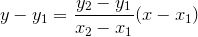The red dot travels around 2 circular arcs, and there are points (the circles) on the pulley wheels that rotate around a center as well.

I needed to use the following parametric equations, where r is the distance from the center to the rotating point, to achieve this: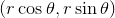As time goes on, the angle θ changes (it's in radians, of course), and the point sweeps out a circle. The starting point is (r, 0) when θ = 0.

## Linear and angular velocities

Of course, the whole point of the applet is to demonstrate the relationship between linear and angular velocity, one of the applications of radian measure. Our basic equation is:

v = rω,

where

v is velocity in meters/second (m/s)

r is radius in meters (m)

I find I understand new formulas more easily when I can see the units.

## Ratios

Pulleys are used to make our lives easier (e.g. gears on a bicycle, or for lifting weights) and they work when there is a difference in the radius between the 2 pulley wheels. So we may need to pedal faster on a bike when in a low gear, but the advantage is we don't have to push so hard.

The applet shows the difference in angular velocity between the larger wheel (lower angular velocity) and the smaller wheel (higher angular velocity).

It turns out the ratio of the angular velocities is the inverse of the ratio of the radii of the 2 wheels.

## Functions

In programming, we can come across 2 kinds of functions:

1. A set of commands that is used over and over (for example there is a function that draws the pulley wheels and the segments, adn this function is called each time you change the radius of a wheel, and the function that moves the red dot and other points around)
2. The mathematical-type function. In this applet, I make use of sine and cosine functions (as well as inverse sine and cosine) to create the parametric equation points I mentioned earlier.

I also needed to use the concept of shifted trigonometric functions (ones where the curve does not start at 0).

## Conclusion

This is actually a relatively simple applet. Programming such applets - and most games - involves a lot of interesting math!

### Comment Preview

HTML: You can use simple tags like <b>, <a href="...">, etc.

To enter math, you can can either:

1. Use simple calculator-like input in the following format (surround your math in backticks, or qq on tablet or phone):
a^2 = sqrt(b^2 + c^2)
(See more on ASCIIMath syntax); or
2. Use simple LaTeX in the following format. Surround your math with $$ and $$.
$$\int g dx = \sqrt{\frac{a}{b}}$$
(This is standard simple LaTeX.)

NOTE: You can mix both types of math entry in your comment.